JEE  >  Test: Vectors- Case Based Type Questions

# Test: Vectors- Case Based Type Questions - JEE

Test Description

## 20 Questions MCQ Test Mathematics (Maths) Class 12 - Test: Vectors- Case Based Type Questions

Test: Vectors- Case Based Type Questions for JEE 2023 is part of Mathematics (Maths) Class 12 preparation. The Test: Vectors- Case Based Type Questions questions and answers have been prepared according to the JEE exam syllabus.The Test: Vectors- Case Based Type Questions MCQs are made for JEE 2023 Exam. Find important definitions, questions, notes, meanings, examples, exercises, MCQs and online tests for Test: Vectors- Case Based Type Questions below.
Solutions of Test: Vectors- Case Based Type Questions questions in English are available as part of our Mathematics (Maths) Class 12 for JEE & Test: Vectors- Case Based Type Questions solutions in Hindi for Mathematics (Maths) Class 12 course. Download more important topics, notes, lectures and mock test series for JEE Exam by signing up for free. Attempt Test: Vectors- Case Based Type Questions | 20 questions in 40 minutes | Mock test for JEE preparation | Free important questions MCQ to study Mathematics (Maths) Class 12 for JEE Exam | Download free PDF with solutions
 1 Crore+ students have signed up on EduRev. Have you?
Test: Vectors- Case Based Type Questions - Question 1

### Direction: Read the following text and answer the following questions on the basis of the same:The cross product of two vectors gives a vector perpendicular to the plane containing the vectors.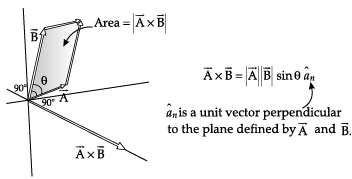Q. If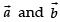are non-zero vectors, then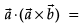Detailed Solution for Test: Vectors- Case Based Type Questions - Question 1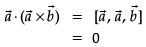Test: Vectors- Case Based Type Questions - Question 2

### Direction: Read the following text and answer the following questions on the basis of the same:The cross product of two vectors gives a vector perpendicular to the plane containing the vectors.Q.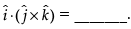Detailed Solution for Test: Vectors- Case Based Type Questions - Question 2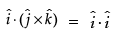= 1

Test: Vectors- Case Based Type Questions - Question 3

### Direction: Read the following text and answer the following questions on the basis of the same:The cross product of two vectors gives a vector perpendicular to the plane containing the vectors.Q.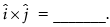Detailed Solution for Test: Vectors- Case Based Type Questions - Question 3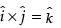Test: Vectors- Case Based Type Questions - Question 4

Direction: Read the following text and answer the following questions on the basis of the same:

The cross product of two vectors gives a vector perpendicular to the plane containing the vectors.Q. The angle between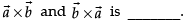Detailed Solution for Test: Vectors- Case Based Type Questions - Question 4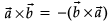The angle between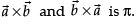Test: Vectors- Case Based Type Questions - Question 5

Direction: Read the following text and answer the following questions on the basis of the same:

The cross product of two vectors gives a vector perpendicular to the plane containing the vectors.Q. Area of parallelogram whose adjacent sides are represented by the vectors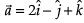and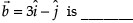sq. units.

Detailed Solution for Test: Vectors- Case Based Type Questions - Question 5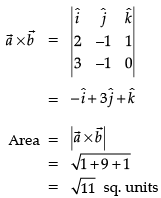Test: Vectors- Case Based Type Questions - Question 6

Direction: Read the following text and answer the following questions on the basis of the same:

A class XII student appearing for a competitive examination was asked to attempt the following questions.

Let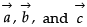be three non zero vectors.

Q. Let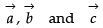be unit vectors such that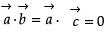and angle between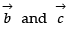is π/6 then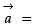Test: Vectors- Case Based Type Questions - Question 7

Direction: Read the following text and answer the following questions on the basis of the same:

A class XII student appearing for a competitive examination was asked to attempt the following questions.

Letbe three non zero vectors.

Q.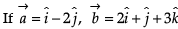then evaluate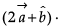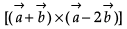Detailed Solution for Test: Vectors- Case Based Type Questions - Question 7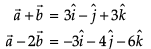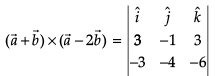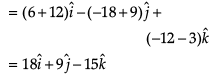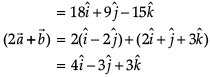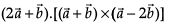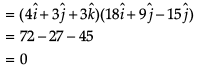Test: Vectors- Case Based Type Questions - Question 8

Direction: Read the following text and answer the following questions on the basis of the same:

A class XII student appearing for a competitive examination was asked to attempt the following questions.

Letbe three non zero vectors.

Q.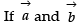are such that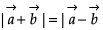then

Test: Vectors- Case Based Type Questions - Question 9

Direction: Read the following text and answer the following questions on the basis of the same:

A class XII student appearing for a competitive examination was asked to attempt the following questions.

Letbe three non zero vectors.

Q.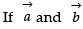are unit vectors and θ be the angle between them then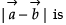Detailed Solution for Test: Vectors- Case Based Type Questions - Question 9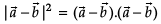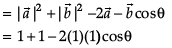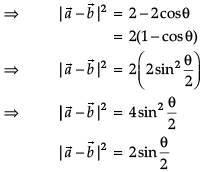Test: Vectors- Case Based Type Questions - Question 10

Direction: Read the following text and answer the following questions on the basis of the same:

A class XII student appearing for a competitive examination was asked to attempt the following questions.

Letbe three non zero vectors.

Q. The area of the parallelogram formed by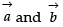as diagonals is

Test: Vectors- Case Based Type Questions - Question 11

Direction: Read the following text and answer the following questions on the basis of the same:

Solar Panels have to be installed carefully so that the tilt of the roof, and the direction to the sun, produce the largest possible electrical power in the solar panels.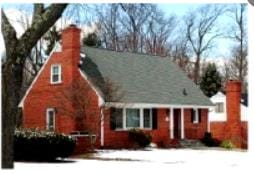A surveyor uses his instrument to determine the coordinates of the four corners of a roof where solar panels are to be mounted. In the picture , suppose the points are labelled counter clockwise from the roof corner nearest to the camera in units of meters P1(6, 8, 4), P2(21, 8, 4), P3(21, 16, 10) and P4(6, 16, 10)

Q. What are the components to the vector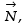perpendicular to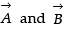and the surface of the roof?

Detailed Solution for Test: Vectors- Case Based Type Questions - Question 11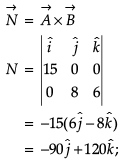Test: Vectors- Case Based Type Questions - Question 12

Direction: Read the following text and answer the following questions on the basis of the same:

Solar Panels have to be installed carefully so that the tilt of the roof, and the direction to the sun, produce the largest possible electrical power in the solar panels.A surveyor uses his instrument to determine the coordinates of the four corners of a roof where solar panels are to be mounted. In the picture , suppose the points are labelled counter clockwise from the roof corner nearest to the camera in units of meters P1(6, 8, 4), P2(21, 8, 4), P3(21, 16, 10) and P4(6, 16, 10)

Q. Write the vector in standard notation with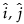and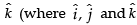are the unit vectors along the three axes).

Test: Vectors- Case Based Type Questions - Question 13

Direction: Read the following text and answer the following questions on the basis of the same:

Solar Panels have to be installed carefully so that the tilt of the roof, and the direction to the sun, produce the largest possible electrical power in the solar panels.A surveyor uses his instrument to determine the coordinates of the four corners of a roof where solar panels are to be mounted. In the picture , suppose the points are labelled counter clockwise from the roof corner nearest to the camera in units of meters P1(6, 8, 4), P2(21, 8, 4), P3(21, 16, 10) and P4(6, 16, 10)

Q. What are the components to the two edge vectors defined by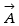= PV of P2 – PV of P1 and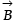= PV of P4 – PV of P1? (where PV stands for position vector)

Detailed Solution for Test: Vectors- Case Based Type Questions - Question 13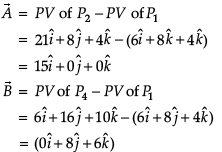∴ A(15, 0,0) and B(0,8,6)

Test: Vectors- Case Based Type Questions - Question 14

Direction: Read the following text and answer the following questions on the basis of the same:

Solar Panels have to be installed carefully so that the tilt of the roof, and the direction to the sun, produce the largest possible electrical power in the solar panels.A surveyor uses his instrument to determine the coordinates of the four corners of a roof where solar panels are to be mounted. In the picture , suppose the points are labelled counter clockwise from the roof corner nearest to the camera in units of meters P1(6, 8, 4), P2(21, 8, 4), P3(21, 16, 10) and P4(6, 16, 10)

Q. What are the magnitudes of the vectors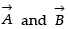and in what units?

Detailed Solution for Test: Vectors- Case Based Type Questions - Question 14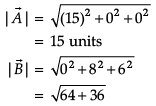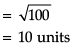Test: Vectors- Case Based Type Questions - Question 15

Direction: Read the following text and answer the following questions on the basis of the same:

Solar Panels have to be installed carefully so that the tilt of the roof, and the direction to the sun, produce the largest possible electrical power in the solar panels.A surveyor uses his instrument to determine the coordinates of the four corners of a roof where solar panels are to be mounted. In the picture , suppose the points are labelled counter clockwise from the roof corner nearest to the camera in units of meters P1(6, 8, 4), P2(21, 8, 4), P3(21, 16, 10) and P4(6, 16, 10)

Q. What is the magnitude of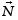and in what units?

Detailed Solution for Test: Vectors- Case Based Type Questions - Question 15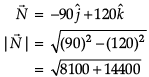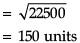Test: Vectors- Case Based Type Questions - Question 16

Direction: Read the following text and answer the following questions on the basis of the same:

ABCD is a parallelogram whose adjacent sides are represented by the vectors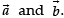Three of its vertices are A(1, 2, 3), B(2, 0, 5) and D(–1, 4, 1).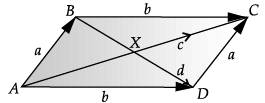Q. The diagonal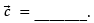Detailed Solution for Test: Vectors- Case Based Type Questions - Question 16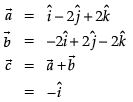Test: Vectors- Case Based Type Questions - Question 17

Direction: Read the following text and answer the following questions on the basis of the same:

ABCD is a parallelogram whose adjacent sides are represented by the vectorsThree of its vertices are A(1, 2, 3), B(2, 0, 5) and D(–1, 4, 1).Q. The vector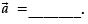Detailed Solution for Test: Vectors- Case Based Type Questions - Question 17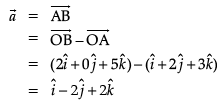Test: Vectors- Case Based Type Questions - Question 18

Direction: Read the following text and answer the following questions on the basis of the same:

ABCD is a parallelogram whose adjacent sides are represented by the vectorsThree of its vertices are A(1, 2, 3), B(2, 0, 5) and D(–1, 4, 1).Q. Area of ABCD = _______ sq. units.

Detailed Solution for Test: Vectors- Case Based Type Questions - Question 18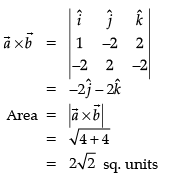Test: Vectors- Case Based Type Questions - Question 19

Direction: Read the following text and answer the following questions on the basis of the same:

ABCD is a parallelogram whose adjacent sides are represented by the vectorsThree of its vertices are A(1, 2, 3), B(2, 0, 5) and D(–1, 4, 1).Q. The length of side CD is _______ units.

Detailed Solution for Test: Vectors- Case Based Type Questions - Question 19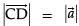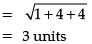Test: Vectors- Case Based Type Questions - Question 20

Direction: Read the following text and answer the following questions on the basis of the same:

ABCD is a parallelogram whose adjacent sides are represented by the vectorsThree of its vertices are A(1, 2, 3), B(2, 0, 5) and D(–1, 4, 1).Q. The diagonal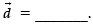Detailed Solution for Test: Vectors- Case Based Type Questions - Question 20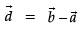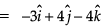## Mathematics (Maths) Class 12

206 videos|264 docs|139 tests
Information about Test: Vectors- Case Based Type Questions Page
In this test you can find the Exam questions for Test: Vectors- Case Based Type Questions solved & explained in the simplest way possible. Besides giving Questions and answers for Test: Vectors- Case Based Type Questions, EduRev gives you an ample number of Online tests for practice

## Mathematics (Maths) Class 12

206 videos|264 docs|139 tests